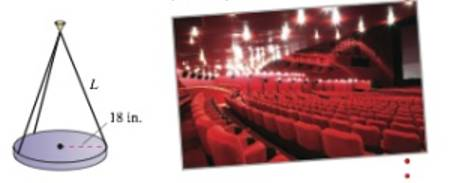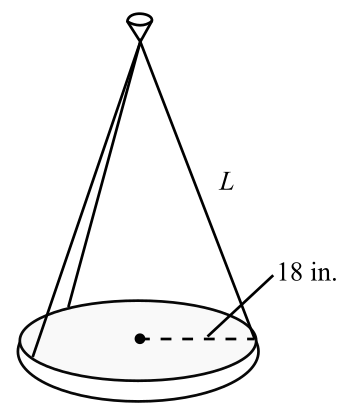Chapter 11.2, Problem 101E

Chapter
Section
Textbook Problem

# Auditorium LightsThe lights in an auditorium are 24-pound discs of radius 18 inches. Each disc is supported by three equally spaced cables that are L inches long (see figure).(a) Write the tension T in each cable as a function of L. Determine the domain of the function.(b) Use a graphing utility and the function in part (a) to complete the table. L 20 25 50 35 40 45 50 T (c) Use a graphing utility to graph the function in pan (a). Determine the asymptotes of the graph.(d) Confirm the asymptotes of the graph in part (c) analytically.(e) Determine the minimum length of each cable when a cable is designed to carry a maximum load of 10 pounds.

(a)

To determine

To calculate: The tension T cable function in the form of L and then determine the domain of the function by considering the given conditions that the lights in an auditorium are 24-pound discs of radius 18 inches and each disc is supported by three equally spaced cables that are L inches long as shown in the figure,Explanation

Given:

The given data is that the lights in an auditorium are 24-pound discs of radius 18 inches and each disc is supported by three equally spaced cables that are L inches long as shown in the figure,

Formula used:

The Pythagorean theorem states that in a right angle triangle if b is the base, h is the height of the triangle then hypotenuse l can be calculated as,

l=b2+h2

Calculation:

Consider the given figure,

From the above figure, can be interpreted,

Consider Q be the origin,

Now in right angle triangle QAP, the hypotenuse l can be found out by,

QA2+AP2=QP2

Here, QA=h,AP=18,QP=l

Thus,

h2+182=l2h=l218

If the coordinate of P is (0,18,0) and Q is (0,0,h), thus,

QP=(0,18,h)

Consider tension be along QP=(0,18,h).

Now the tension vector T in each wire is,

T=c(0,18,h)

Since the weight is vertically in down direction therefore the other components are zero again all the three strings are of equal length so each one has the equal tension that is 243=8pounds

(b)

To determine

To calculate: The complete table of the function T=8LL2182 with the help of graphing utility and the

table is given as,

 L 20 25 30 35 40 45 50 T

(c)

To determine

To graph: The function T=8LL2182 and then determine the asymptotes of the graph.

(d)

To determine

To prove: The asymptotes L=18 and T=8 obtained in part (c) is analytically correct.

(e)

To determine

To calculate: The minimum length of each cable when a cable is designed to lift a maximum load of 10pounds if the function is T=8LL2182.

### Still sussing out bartleby?

Check out a sample textbook solution.

See a sample solution

#### The Solution to Your Study Problems

Bartleby provides explanations to thousands of textbook problems written by our experts, many with advanced degrees!

Get Started

#### Find more solutions based on key concepts